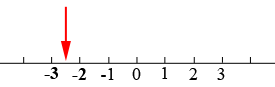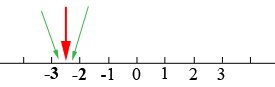SEARCH HOMEMath Central Quandaries & QueriesQuestion from Medha: Find 12 rational numbers between -2 and -3Hi,

First draw a number line and mark on it -2 and -3.

Step 1:

Find a rational number between -2 and -3.Step 2:

Find a rational number between the one you just found in step 1 and -2 and then another rational number between the one you found in step 1 and -3.Continue.

PennyMath Central is supported by the University of Regina and The Pacific Institute for the Mathematical Sciences.